# Numeral Systems Numeral System Positional systems Decimal Binary

• Slides: 32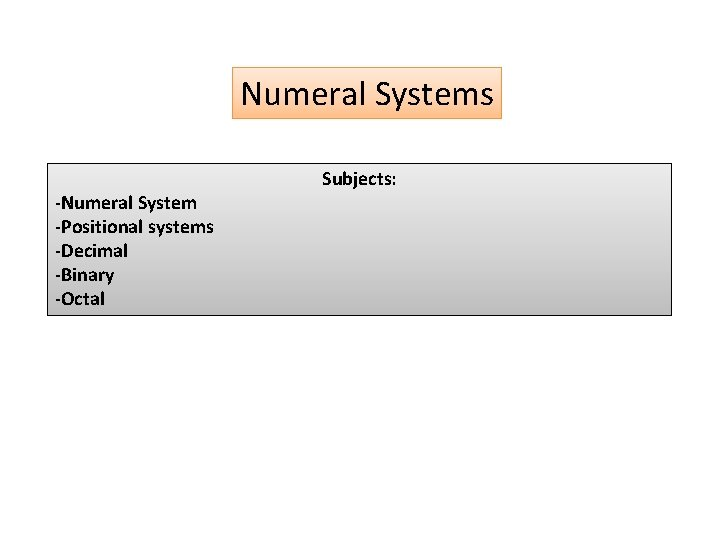Numeral Systems -Numeral System -Positional systems -Decimal -Binary -Octal Subjects:Introduction A numeral system (or system of numeration) is a writing system for expressing numbers, that is a mathematical notation for representing numbers of a given set, using digits or other symbols in a consistent manner. It can be seen as the context that allows the symbols "11" to be interpreted as the binary symbol for three, the decimal symbol for eleven, or a symbol for other numbers in different bases. Ideally, a numeral system will: q. Represent a useful set of numbers (e. g. all integers, or rational numbers); q. Give every number represented a unique representation (or at least a standard representation); q. Reflect the algebraic and arithmetic structure of the numbers. ü A number is a mathematical object used to count, label, and measure. ü A digit is a symbol (a numeral symbol such as "3" or "7") used in combinations (such as "37") to represent numbers in positional numeral systems. The ten digits of the Western Arabic numerals, in order of value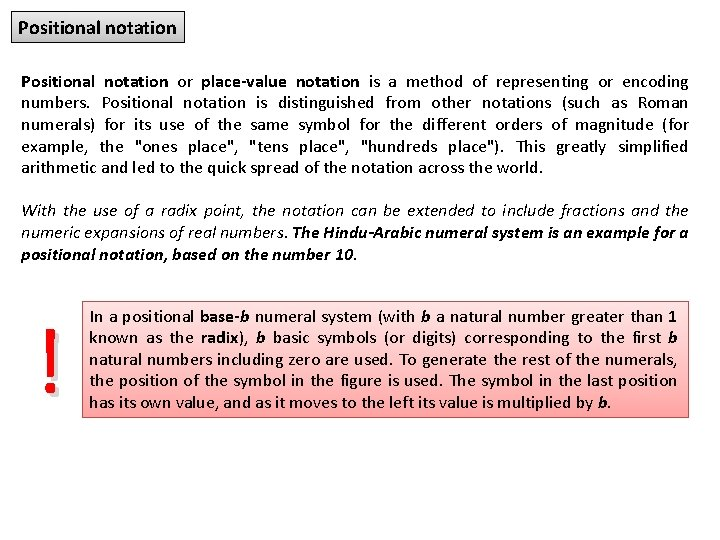Positional notation or place-value notation is a method of representing or encoding numbers. Positional notation is distinguished from other notations (such as Roman numerals) for its use of the same symbol for the different orders of magnitude (for example, the "ones place", "tens place", "hundreds place"). This greatly simplified arithmetic and led to the quick spread of the notation across the world. With the use of a radix point, the notation can be extended to include fractions and the numeric expansions of real numbers. The Hindu-Arabic numeral system is an example for a positional notation, based on the number 10. ! In a positional base-b numeral system (with b a natural number greater than 1 known as the radix), b basic symbols (or digits) corresponding to the first b natural numbers including zero are used. To generate the rest of the numerals, the position of the symbol in the figure is used. The symbol in the last position has its own value, and as it moves to the left its value is multiplied by b.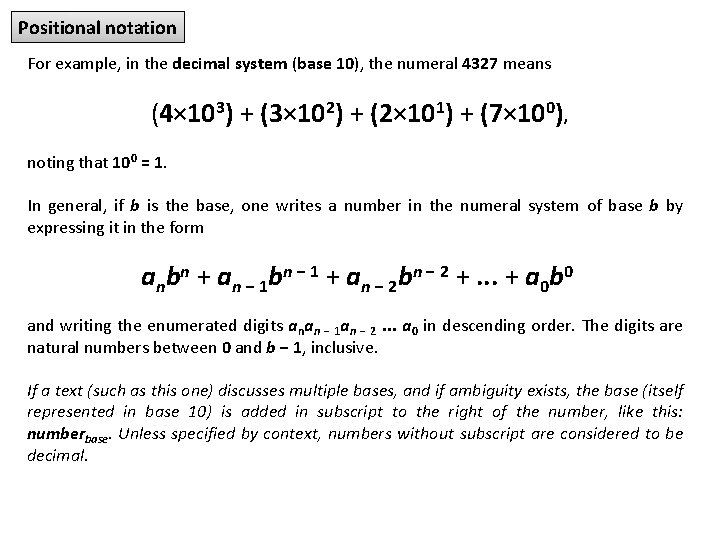Positional notation For example, in the decimal system (base 10), the numeral 4327 means (4× 103) + (3× 102) + (2× 101) + (7× 100), noting that 100 = 1. In general, if b is the base, one writes a number in the numeral system of base b by expressing it in the form anbn + an − 1 bn − 1 + an − 2 bn − 2 +. . . + a 0 b 0 and writing the enumerated digits anan − 1 an − 2. . . a 0 in descending order. The digits are natural numbers between 0 and b − 1, inclusive. If a text (such as this one) discusses multiple bases, and if ambiguity exists, the base (itself represented in base 10) is added in subscript to the right of the number, like this: numberbase. Unless specified by context, numbers without subscript are considered to be decimal.Positional notation By using a dot to divide the digits into two groups, one can also write fractions in the positional system. For example, the base-2 numeral 10. 11 denotes 1× 21 + 0× 20 + 1× 2− 1 + 1× 2− 2 = 2. 75. In general, numbers in the base b system are of the form: The numbers bk and b−k are the weights of the corresponding digits. The position k is the logarithm of the corresponding weight w, that is The number of tally marks required in the unary numeral system for describing the weight would have been w. In the positional system, the number of digits required to describe it is only for .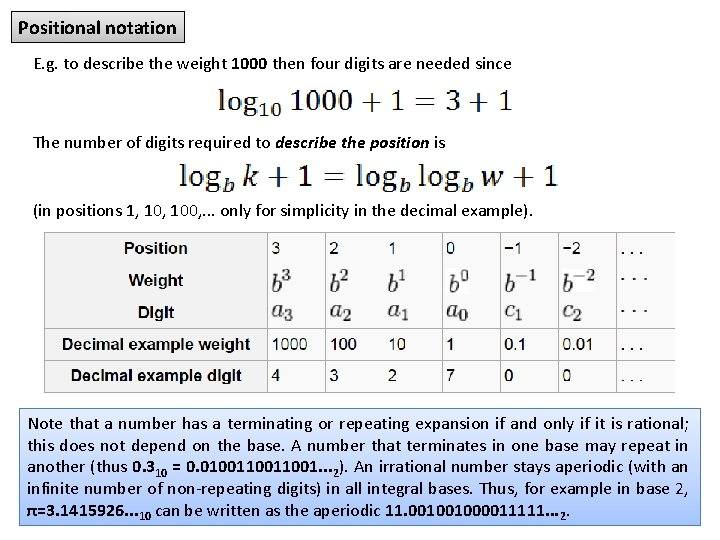Positional notation E. g. to describe the weight 1000 then four digits are needed since The number of digits required to describe the position is (in positions 1, 100, . . . only for simplicity in the decimal example). Note that a number has a terminating or repeating expansion if and only if it is rational; this does not depend on the base. A number that terminates in one base may repeat in another (thus 0. 310 = 0. 010011001. . . 2). An irrational number stays aperiodic (with an infinite number of non-repeating digits) in all integral bases. Thus, for example in base 2, π=3. 1415926. . . 10 can be written as the aperiodic 11. 001001000011111. . . 2.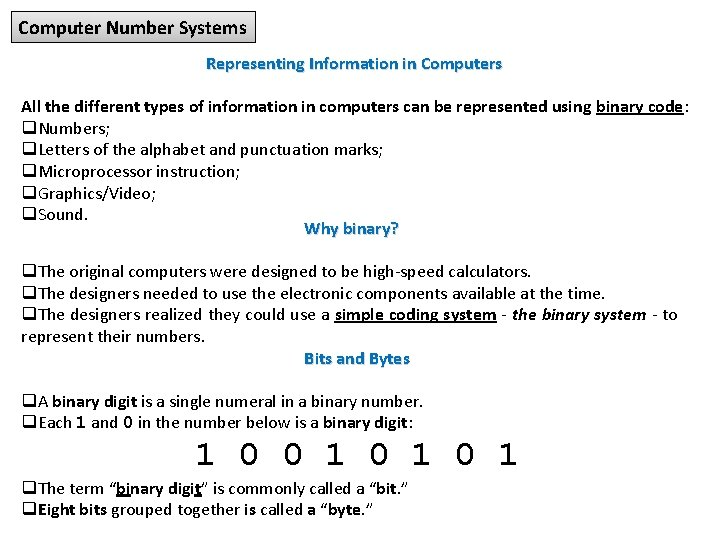Computer Number Systems Representing Information in Computers All the different types of information in computers can be represented using binary code: q. Numbers; q. Letters of the alphabet and punctuation marks; q. Microprocessor instruction; q. Graphics/Video; q. Sound. Why binary? q. The original computers were designed to be high-speed calculators. q. The designers needed to use the electronic components available at the time. q. The designers realized they could use a simple coding system - the binary system - to represent their numbers. Bits and Bytes q. A binary digit is a single numeral in a binary number. q. Each 1 and 0 in the number below is a binary digit: 1 0 0 1 0 1 q. The term “binary digit” is commonly called a “bit. ” q. Eight bits grouped together is called a “byte. ”Computer Number Systems are: q. Decimal Numbers q. Binary Numbers q. Hexadecimal Numbers The study of number systems is useful due to the fact that number systems other than the familiar decimal (base 10) number system are used in the computer field. Digital computers internally use the binary (base 2) number system to represent data and perform arithmetic calculations. The binary number system is very efficient for computers, but not for humans. Representing even relatively small numbers with the binary system requires working with long strings of ones and zeroes. The hexadecimal (base 16) number system (often called "hex" for short) provides us with a shorthand method of working with binary numbers. One digit in hex corresponds to four binary digits (bits), so the internal representation of one byte can be represented either by eight binary digits or two hexadecimal digits. Less commonly used is the octal (base 8) number system, where one digit in octal corresponds to three binary digits (bits).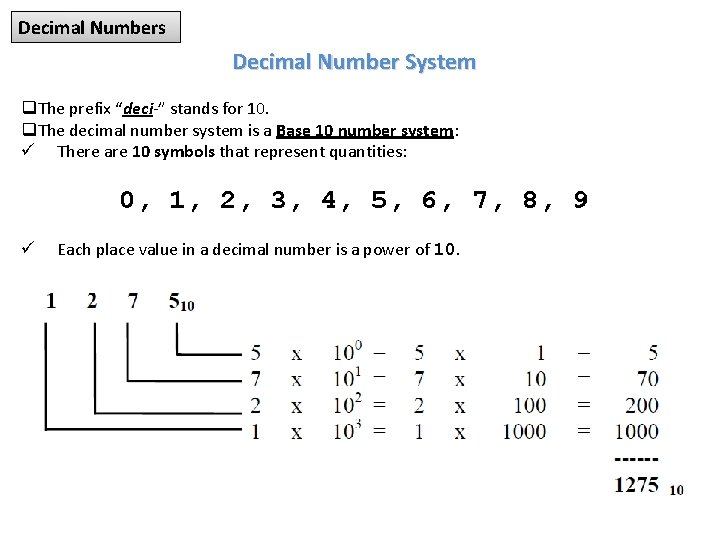Decimal Numbers Decimal Number System q. The prefix “deci-” stands for 10. q. The decimal number system is a Base 10 number system: ü There are 10 symbols that represent quantities: 0, 1, 2, 3, 4, 5, 6, 7, 8, 9 ü Each place value in a decimal number is a power of 10.Binary Numbers The Binary Number System q. The prefix “bi-” stands for 2. q. The binary number system is a Base 2 number system: ü There are 2 symbols that represent quantities: 0, 1 ü Each place value in a binary number is a power of 2. From right to left, the successive positions of the binary number are weighted 1, 2, 4, 8, 16, 32, 64, etc. A list of the first several powers of 2 follows:Binary Numbers For reference, the following table shows the decimal numbers 0 through 31 with their binary equivalents: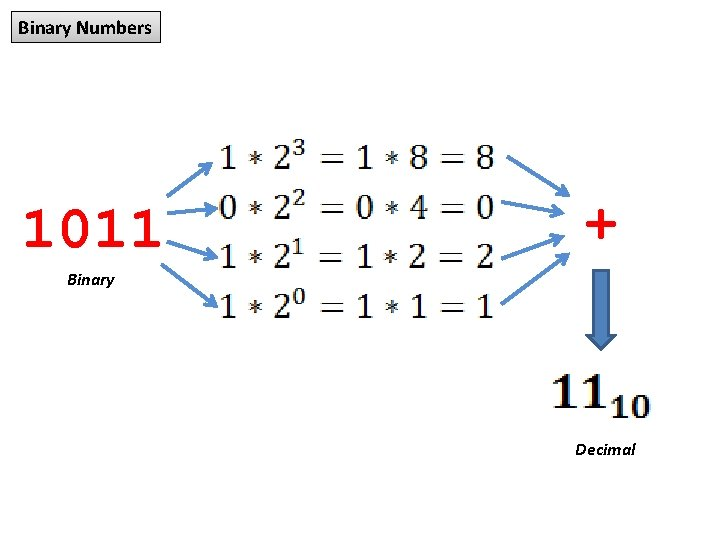Binary Numbers 1011 + Binary DecimalConverting a Binary Number to a Decimal Number To determine the value of a binary number (1001, for example), we can expand the number using the positional weights as follows: Here's another example to determine the value of the binary number 1101010:Converting a Decimal Number to a Binary Number To convert a decimal number to its binary equivalent, the remainder method can be used. (This method can be used to convert a decimal number into any other base. ) The remainder method involves the following four steps: ! 1) Divide the decimal number by the base (in the case of binary, divide by 2). 2) Indicate the remainder to the right. 3) Continue dividing into each quotient (and indicating the remainder) until the divide operation produces a zero quotient. 4) The base 2 number is the numeric remainder reading from the last division to the first (if you start at the bottom, the answer will read from top to bottom).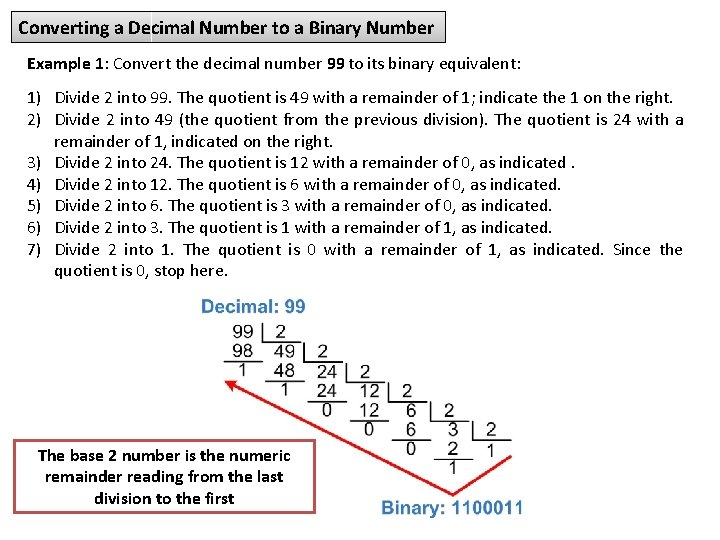Converting a Decimal Number to a Binary Number Example 1: Convert the decimal number 99 to its binary equivalent: 1) Divide 2 into 99. The quotient is 49 with a remainder of 1; indicate the 1 on the right. 2) Divide 2 into 49 (the quotient from the previous division). The quotient is 24 with a remainder of 1, indicated on the right. 3) Divide 2 into 24. The quotient is 12 with a remainder of 0, as indicated. 4) Divide 2 into 12. The quotient is 6 with a remainder of 0, as indicated. 5) Divide 2 into 6. The quotient is 3 with a remainder of 0, as indicated. 6) Divide 2 into 3. The quotient is 1 with a remainder of 1, as indicated. 7) Divide 2 into 1. The quotient is 0 with a remainder of 1, as indicated. Since the quotient is 0, stop here. The base 2 number is the numeric remainder reading from the last division to the first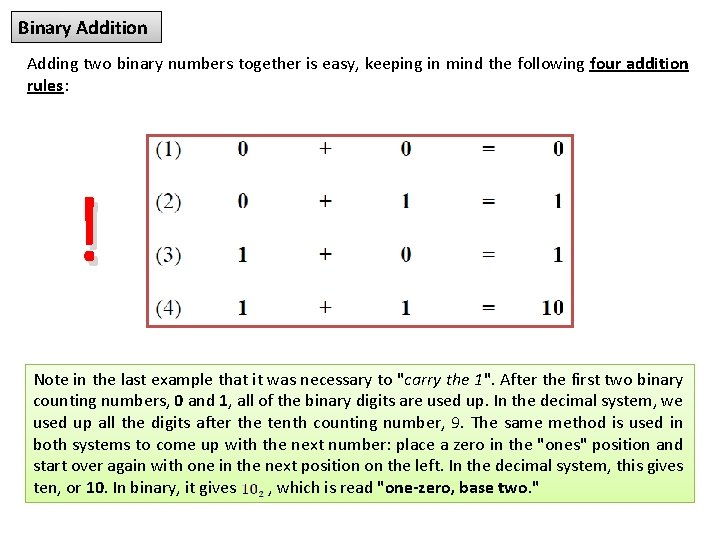Binary Addition Adding two binary numbers together is easy, keeping in mind the following four addition rules: ! Note in the last example that it was necessary to "carry the 1". After the first two binary counting numbers, 0 and 1, all of the binary digits are used up. In the decimal system, we used up all the digits after the tenth counting number, 9. The same method is used in both systems to come up with the next number: place a zero in the "ones" position and start over again with one in the next position on the left. In the decimal system, this gives ten, or 10. In binary, it gives , which is read "one-zero, base two. "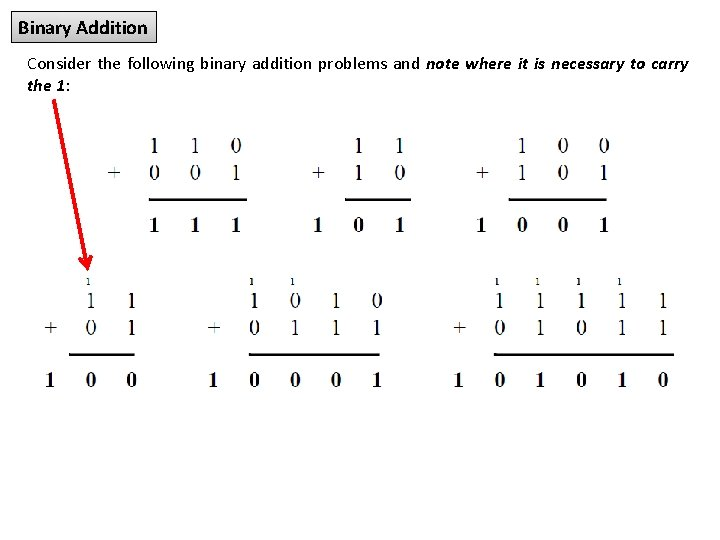Binary Addition Consider the following binary addition problems and note where it is necessary to carry the 1: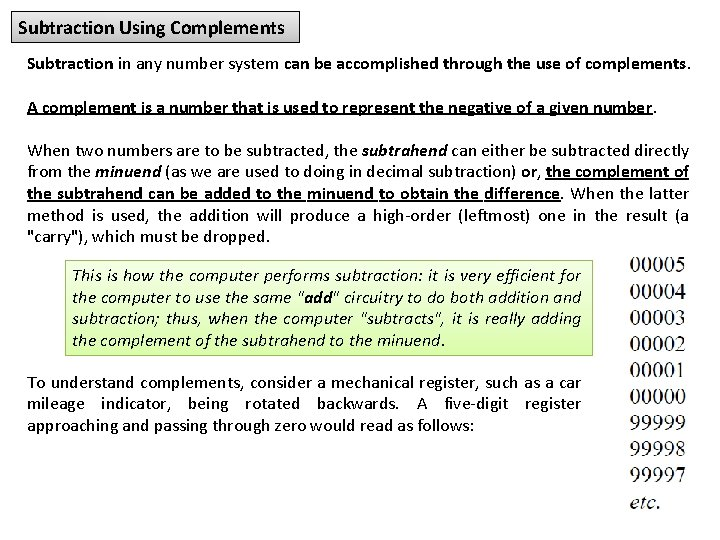Subtraction Using Complements Subtraction in any number system can be accomplished through the use of complements. A complement is a number that is used to represent the negative of a given number. When two numbers are to be subtracted, the subtrahend can either be subtracted directly from the minuend (as we are used to doing in decimal subtraction) or, the complement of the subtrahend can be added to the minuend to obtain the difference. When the latter method is used, the addition will produce a high-order (leftmost) one in the result (a "carry"), which must be dropped. This is how the computer performs subtraction: it is very efficient for the computer to use the same "add" circuitry to do both addition and subtraction; thus, when the computer "subtracts", it is really adding the complement of the subtrahend to the minuend. To understand complements, consider a mechanical register, such as a car mileage indicator, being rotated backwards. A five-digit register approaching and passing through zero would read as follows: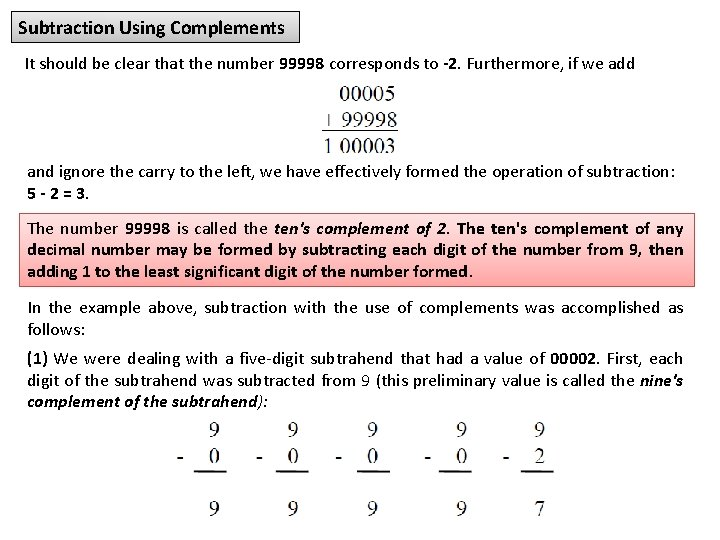Subtraction Using Complements It should be clear that the number 99998 corresponds to -2. Furthermore, if we add and ignore the carry to the left, we have effectively formed the operation of subtraction: 5 - 2 = 3. The number 99998 is called the ten's complement of 2. The ten's complement of any decimal number may be formed by subtracting each digit of the number from 9, then adding 1 to the least significant digit of the number formed. In the example above, subtraction with the use of complements was accomplished as follows: (1) We were dealing with a five-digit subtrahend that had a value of 00002. First, each digit of the subtrahend was subtracted from 9 (this preliminary value is called the nine's complement of the subtrahend):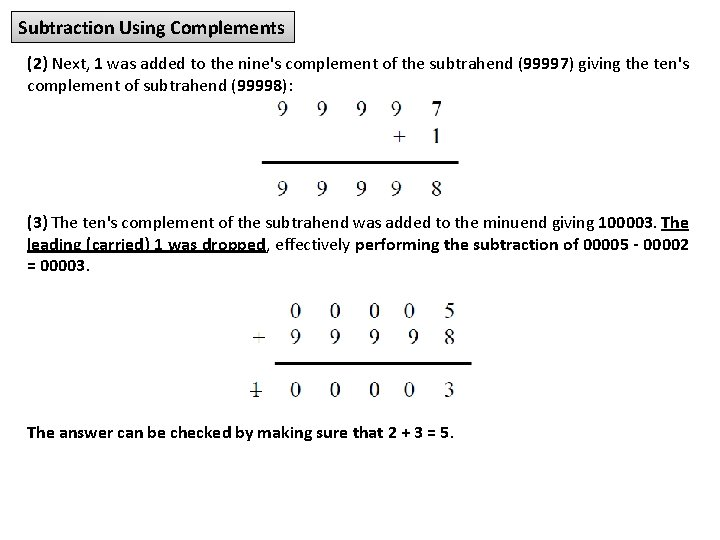Subtraction Using Complements (2) Next, 1 was added to the nine's complement of the subtrahend (99997) giving the ten's complement of subtrahend (99998): (3) The ten's complement of the subtrahend was added to the minuend giving 100003. The leading (carried) 1 was dropped, effectively performing the subtraction of 00005 - 00002 = 00003. The answer can be checked by making sure that 2 + 3 = 5.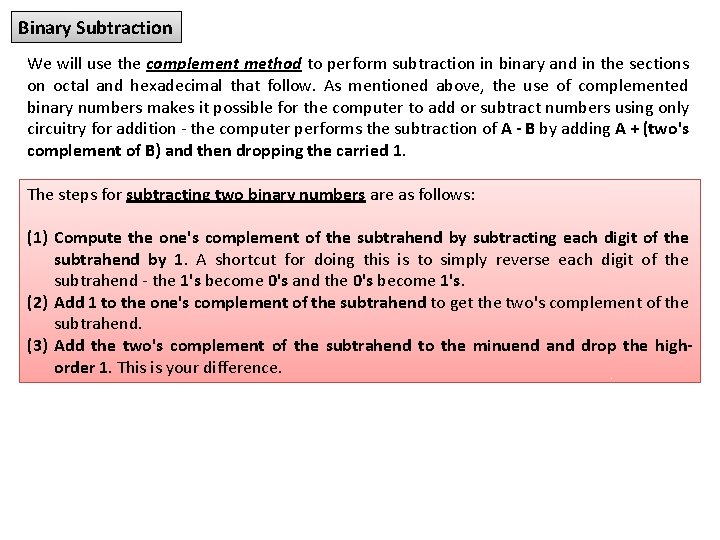Binary Subtraction We will use the complement method to perform subtraction in binary and in the sections on octal and hexadecimal that follow. As mentioned above, the use of complemented binary numbers makes it possible for the computer to add or subtract numbers using only circuitry for addition - the computer performs the subtraction of A - B by adding A + (two's complement of B) and then dropping the carried 1. The steps for subtracting two binary numbers are as follows: (1) Compute the one's complement of the subtrahend by subtracting each digit of the subtrahend by 1. A shortcut for doing this is to simply reverse each digit of the subtrahend - the 1's become 0's and the 0's become 1's. (2) Add 1 to the one's complement of the subtrahend to get the two's complement of the subtrahend. (3) Add the two's complement of the subtrahend to the minuend and drop the highorder 1. This is your difference.Binary Subtraction Example 1: Compute 11010101 – 1001011 (1) Compute the one's complement of 1001011 by subtracting each digit from 1 (note that a leading zero was added to the 7 -digit subtrahend to make it the same size as the 8 -digit minuend): (Note that the one's complement of the subtrahend causes each of the original digits to be reversed. ) (2) Add 1 to the one's complement of the subtrahend, giving the two's complement of the subtrahend: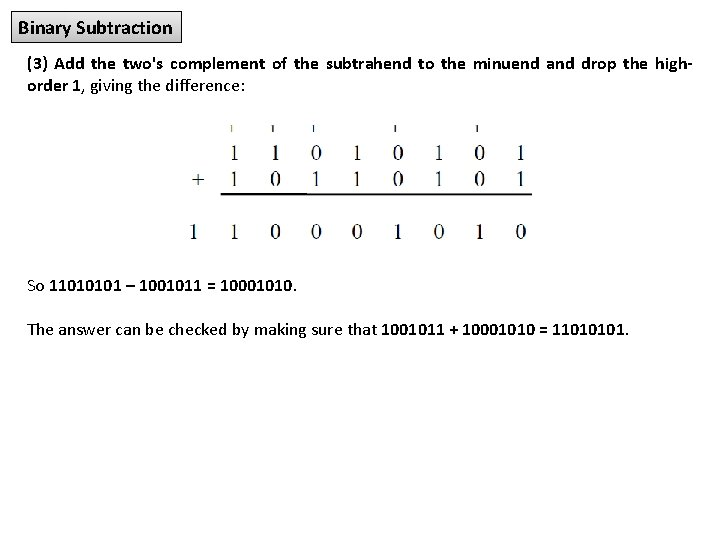Binary Subtraction (3) Add the two's complement of the subtrahend to the minuend and drop the highorder 1, giving the difference: So 11010101 – 1001011 = 10001010. The answer can be checked by making sure that 1001011 + 10001010 = 11010101.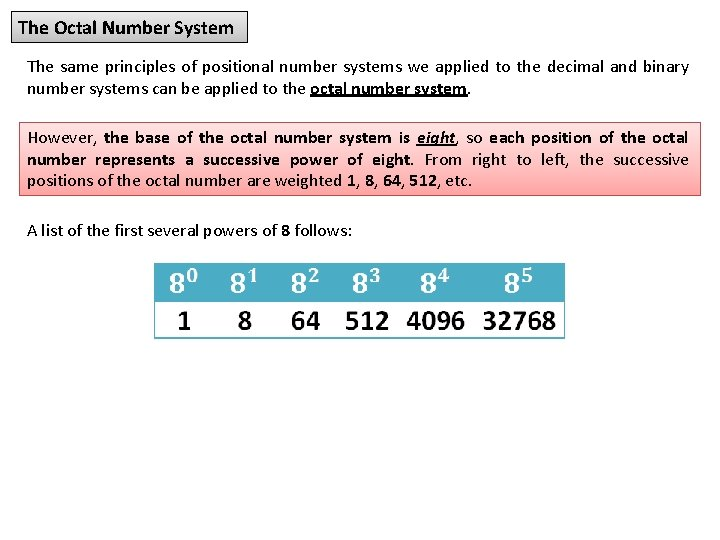The Octal Number System The same principles of positional number systems we applied to the decimal and binary number systems can be applied to the octal number system. However, the base of the octal number system is eight, so each position of the octal number represents a successive power of eight. From right to left, the successive positions of the octal number are weighted 1, 8, 64, 512, etc. A list of the first several powers of 8 follows: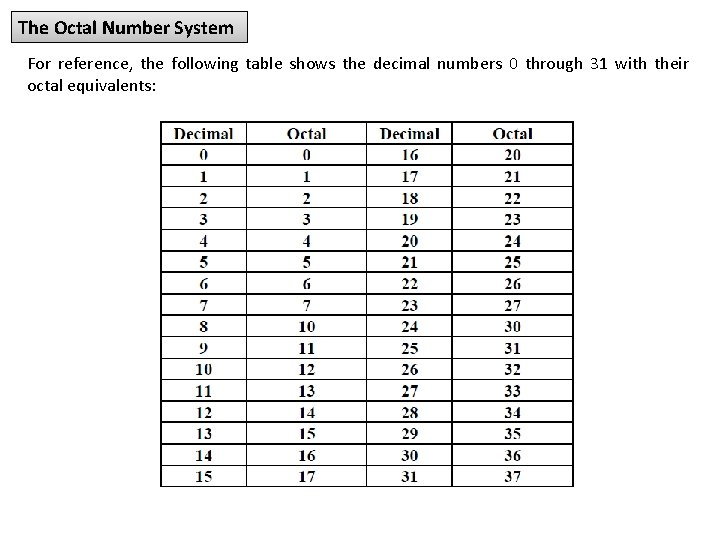The Octal Number System For reference, the following table shows the decimal numbers 0 through 31 with their octal equivalents: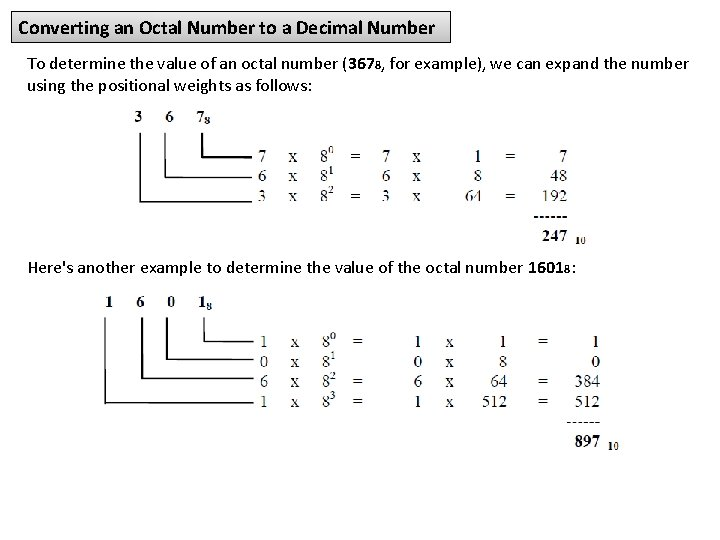Converting an Octal Number to a Decimal Number To determine the value of an octal number (3678, for example), we can expand the number using the positional weights as follows: Here's another example to determine the value of the octal number 16018: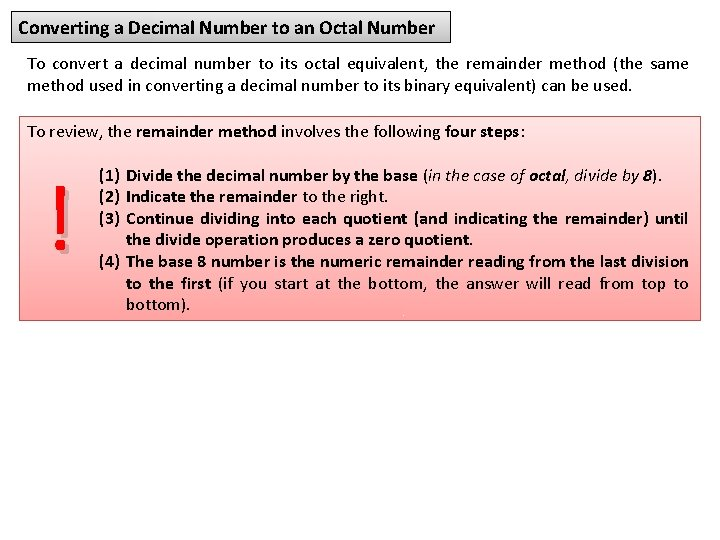Converting a Decimal Number to an Octal Number To convert a decimal number to its octal equivalent, the remainder method (the same method used in converting a decimal number to its binary equivalent) can be used. To review, the remainder method involves the following four steps: ! (1) Divide the decimal number by the base (in the case of octal, divide by 8). (2) Indicate the remainder to the right. (3) Continue dividing into each quotient (and indicating the remainder) until the divide operation produces a zero quotient. (4) The base 8 number is the numeric remainder reading from the last division to the first (if you start at the bottom, the answer will read from top to bottom).Converting a Decimal Number to an Octal Number Example 1: Convert the decimal number 46510 to its octal equivalent: 1) Divide 8 into 465. The quotient is 58 with a remainder of 1; indicate the 1 on the right. 2) Divide 8 into 58 (the quotient from the previous division). The quotient is 7 with a remainder of 2, indicated on the right. 3) Divide 8 into 7. The quotient is 0 with a remainder of 7, as indicated. Since the quotient is 0, stop here. The answer, reading the remainders from top to bottom, is 721, so 46510 = 7218.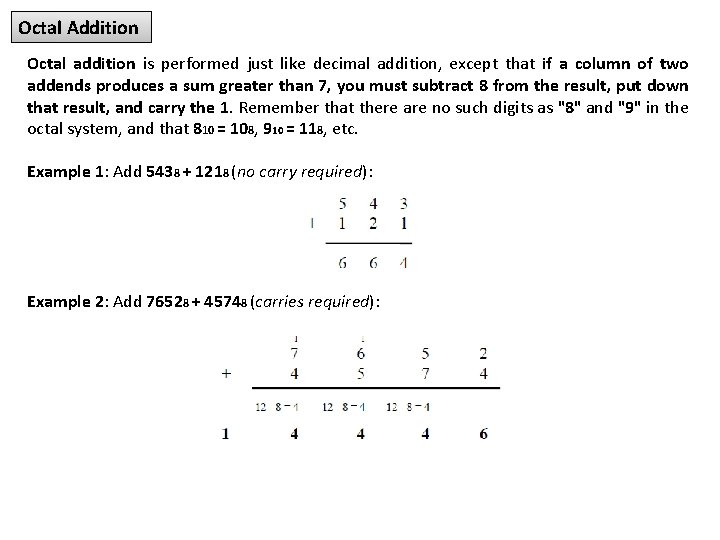Octal Addition Octal addition is performed just like decimal addition, except that if a column of two addends produces a sum greater than 7, you must subtract 8 from the result, put down that result, and carry the 1. Remember that there are no such digits as "8" and "9" in the octal system, and that 810 = 108, 910 = 118, etc. Example 1: Add 5438 + 1218 (no carry required): Example 2: Add 76528 + 45748 (carries required):Octal Subtraction We will use the complement method to perform octal subtraction. The steps for subtracting two octal numbers are as follows: (1) Compute the seven's complement of the subtrahend by subtracting each digit of the subtrahend by 7. (2) Add 1 to the seven's complement of the subtrahend to get the eight's complement of the subtrahend. (3) Add the eight's complement of the subtrahend to the minuend and drop the highorder 1. This is your difference. Example 1: Compute 75268 - 31428 (1) Compute the seven's complement of 31428 by subtracting each digit from 7: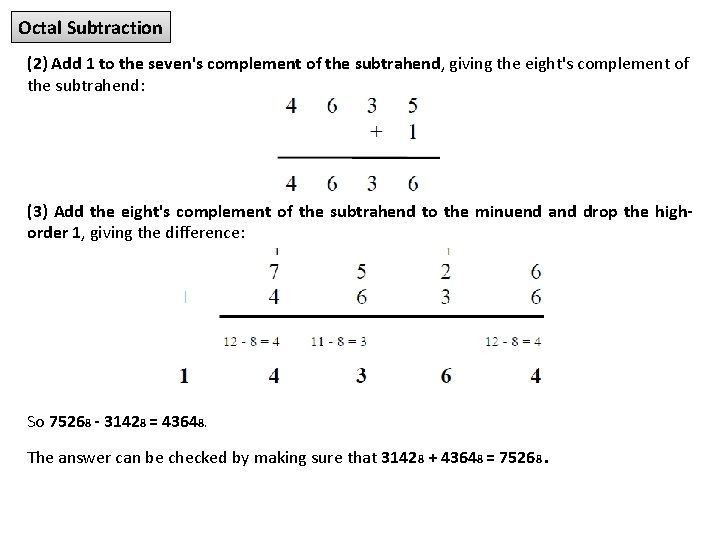Octal Subtraction (2) Add 1 to the seven's complement of the subtrahend, giving the eight's complement of the subtrahend: (3) Add the eight's complement of the subtrahend to the minuend and drop the highorder 1, giving the difference: So 75268 - 31428 = 43648. The answer can be checked by making sure that 31428 + 43648 = 75268.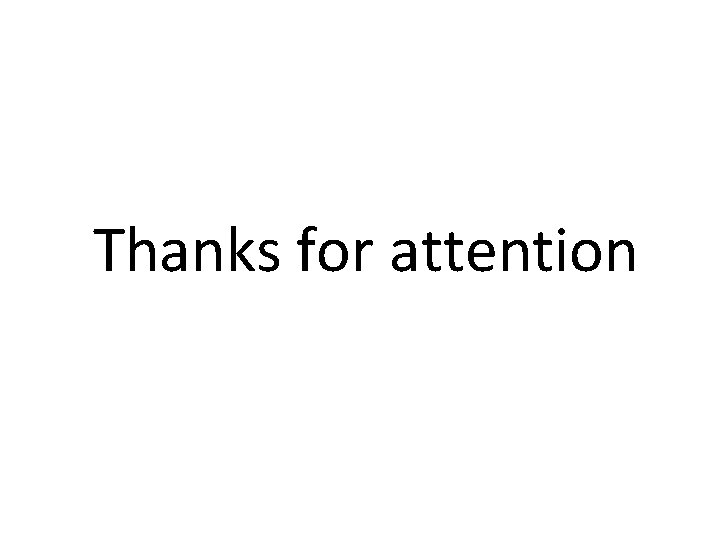Thanks for attention